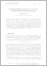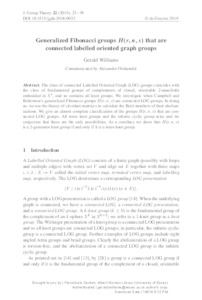# Generalized Fibonacci groups H(r,n,s) that are connected labelled oriented graph groups

Williams, Gerald (2019) 'Generalized Fibonacci groups H(r,n,s) that are connected labelled oriented graph groups.' Journal of Group Theory, 22 (1). 23 - 39. ISSN 1433-5883Preview
Text
[Journal of Group Theory] Generalized Fibonacci groups H(rns) that are connected labelled oriented graph groups.pdf - Published Version

Download (238kB) | Preview

## Abstract

The class of connected Labelled Oriented Graph (LOG) groups coincides with the class of fundamental groups of complements of closed, orientable 2-manifolds embedded in S⁴, and so contains all knot groups. We investigate when Campbell and Robertson’s generalized Fibonacci groups H(r,n,s) are connected LOG groups. In doing so, we use the theory of circulant matrices to calculate the Betti numbers of their abelianizations. We give an almost complete classification of the groups H(r,n,s) that are connected LOG groups. All torus knot groups and the infinite cyclic group arise and we conjecture that these are the only possibilities. As a corollary we show that H(r,n,s) is a 2-generator knot group if and only if it is a torus knot group.

Item Type: Article Q Science > QA Mathematics Faculty of Science and Health > Mathematical Sciences, Department of Elements 08 Jan 2019 16:27 13 Nov 2019 11:15 http://repository.essex.ac.uk/id/eprint/23741

### Actions (login required)View Item